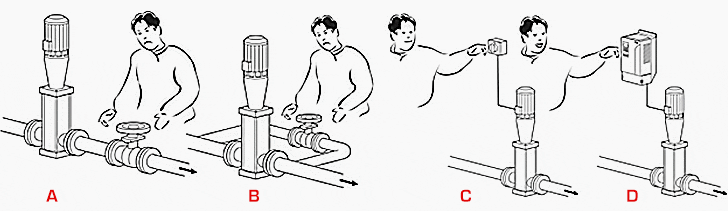﻿ Comparison of 4 Different Flow Control Methods Of Pumps :Back

## Comparison of 4 Different Flow Control Methods Of Pumps :# Comparison of 4 Different Flow Control Methods Of Pumps

## Pump as a part of the process

When in use, the pumps are always part of a pumping system. A pumping system is usually a network of pipes, tanks, valves and other system parts. The receiver is usually at a higher geographic level than the supply of the system. These parts can be also on the same level, as in the case of a closed circuit heat transfer system.

Pumping systems nearly always require a variation of flow rate.

Examples include the daily cycle in the consumption of drinking water, the varying process demand for a liquid or seasonal heating demand. However, the variation required may be in the pump head, such as for cyclical changes in process pressure, or pumping to tanks with a variable liquid level.

In spite of the variations, the pump capacity is selected according to the maximum flow and head or even to the future needs, perhaps with a certain safety margin.

The average pumping capacity may be only a fraction of the maximum capacity and this will require some kind of control.

## Flow control methods

There are several different methods to match the flow to the system requirements. The four most common flow control methods of pumps are throttling, bypassing, on-off control and variable speed drive (VSD) control. These are illustrated in Figure 1.Figure 1 – Illustrations of pump flow control methods. A – throttling, B – bypassing, C – on-off control and D – VSD control

The relative power consumption of the different control methods can be estimated from the area between the x and y-axes and the operating point.

It is using the formula:

P = Q x H

In the following example (see Figure 2), the relative power consumption on an average flow rate of 70% is calculated with different control methods. More detailed explanations on power consumption and energy savings relating to different pump applications are described in the following chapters.

Power consumption of 4 flow control methods

 Control Energy Throttling 89 Bypassing 82 On-off control 70 VSD control 45

### Throttling :

Throttle control is the most commonly used method. The flow caused by the constant speed pump is reduced by increasing the losses in the system by closing the valve. In the example in Figure 2 the operating point is moved from (Q = 10, H = 10) to (Q = 7, H = 12.7).

The relative power consumption can be calculated by:

P = 7 x 12.7 = 89

### Bypassing :

Although not commonly used, bypassing is applied mainly to circulation pumps. The flow output to the system is reduced by bypassing part of the pump discharge flow to the pump suction. This means that the total flow increases (from 10 to 12.4), but the head decreases (from 10 to 6.6).

The relative power consumption is:

P = 12.4 x 6.6 = 82

### On-off control :

On-off control is often used where stepless control is not necessary, such as keeping the pressure in a tank between preset limits. The pump is either running or stopped. The average flow is the relationship between the “on” time and the “total” time (on+off).

The relative power consumption can be easily calculated by:

P = 0.7 x 100 = 70

### VSD control :

To understand the benefits of VSD control consider to the pump curves in Figure 2. With low static head systems, the optimal efficiency of the pump follows the system curve. With VSD control, the duty point of the pump follows the unchanged system curve.

Changing the speed of the pump moves the pump curves in accordance with the affinity laws. If the pump impeller speed is reduced, the pump curve moves downwards. If the speed is increased, it moves upwards. This means that the pumping capacity is exactly matched to the process requirements. According to our earlier example both flow rate (from 10 to 7) and head (from 10 to 6.4) are reduced.

The relative power consumption can be calculated by: P = 7 x 6.4 = 45

This example shows that the variable speed control method is the most energy efficient for pumping applications. The examples discussed were calculated for one flow rate only (70%), but the relative power consumption with different control methods depends on the flow rate. This relationship is shown in Figure 3 (see below).

In these curves, the pump, motor and drive efficiencies are also taken into account and for that reason the results differ somewhat to those in Figure 2.

Throttling control leads to high loss in the pump and in the valve when the system is running at a reduced flow rate. The loss in the motor remains relatively constant over the whole flow range. In VSD control, the operating point follows the system curve, which is optimal for pump efficiency. In general, based on affinity laws, the energy consumption drops dramatically when speed is reduced.

The energy savings with variable speed drive (VSD) controlare significant.